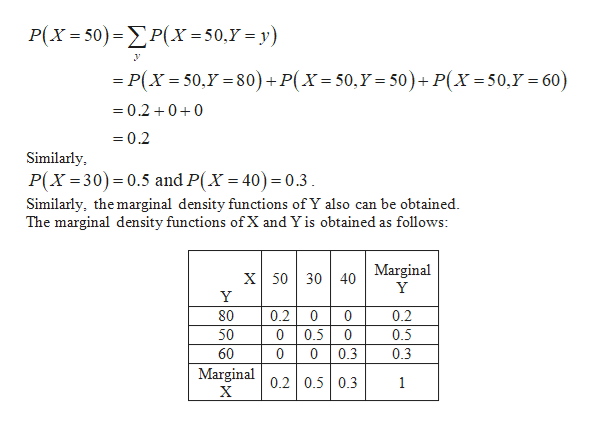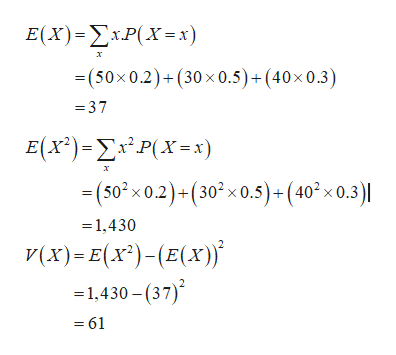# My last question I mixed the data and the question, my bad!5) Given below is a bivariate distribution for the random variables x and y.f(x, y)xy0.250800.530500.34060Compute the expected value and the variance for x and y.Develop a probability distribution for x + y.Using the result of part (b), compute E(x + y) and Var(x + y).Compute the covariance and correlation for x and y. Are x and y positively related, negatively related, or unrelated?Is the variance of the sum of x and y bigger, smaller, or the same as the sum of the individual variances? Why?

Question
187 views

My last question I mixed the data and the question, my bad!

5) Given below is a bivariate distribution for the random variables x and y.

 f(x, y) x y 0.2 50 80 0.5 30 50 0.3 40 60
1. Compute the expected value and the variance for x and y.
2. Develop a probability distribution for x + y.
3. Using the result of part (b), compute E(x + y) and Var(x + y).
4. Compute the covariance and correlation for x and y. Are x and y positively related, negatively related, or unrelated?
5. Is the variance of the sum of x and y bigger, smaller, or the same as the sum of the individual variances? Why?
check_circle

star
star
star
star
star
1 Rating
Step 1

Note:

Hi! Thank you for posting the question. Since your question has more than 3 parts, we have solved the first 3 parts for you. If you need help with any of the other parts, please re-post the question and mention the part you need help with.

Step 2

The marginal density function of X is obtained as follows:help_outlineImage TranscriptioncloseP(X 50) P(x =50,Y = y) -P(X 50,Y 80)+P(X- 50,Y= 50)+ P(X=50,Y 60) 0.2 00 =0.2 Similarly P(X 30) 0.5 and P(X = 40) 0.3 Similarly, the marginal density functions of Y also can be obtained. The marginal density functions of X and Y is obtained as follows: X 5030 40 Marginal Y Y 0.2 80 0.2 0 0 50 0.5 0 0.3 Marginal 02 0.5 0.3 0.5 0 0 60 0 0.3 1 X fullscreen
Step 3

1. Finding the expected value and the variance of X and Y:

The expected value and the variance of X are 37...help_outlineImage TranscriptioncloseE (X)-ΣxΡ(X=x) (50x0.2)+(30x 0.5)+(40x0.3) =37 E()-ΣP(X= . -(50x 0.2)+(30x 0.5)+(40 x 0.3) х 1,430 γ(x) - E(x) - (E(χ)} 1,430- (37) = 61 fullscreen

### Want to see the full answer?

See Solution

#### Want to see this answer and more?

Solutions are written by subject experts who are available 24/7. Questions are typically answered within 1 hour.*

See Solution
*Response times may vary by subject and question.
Tagged in
MathStatistics

### Other